#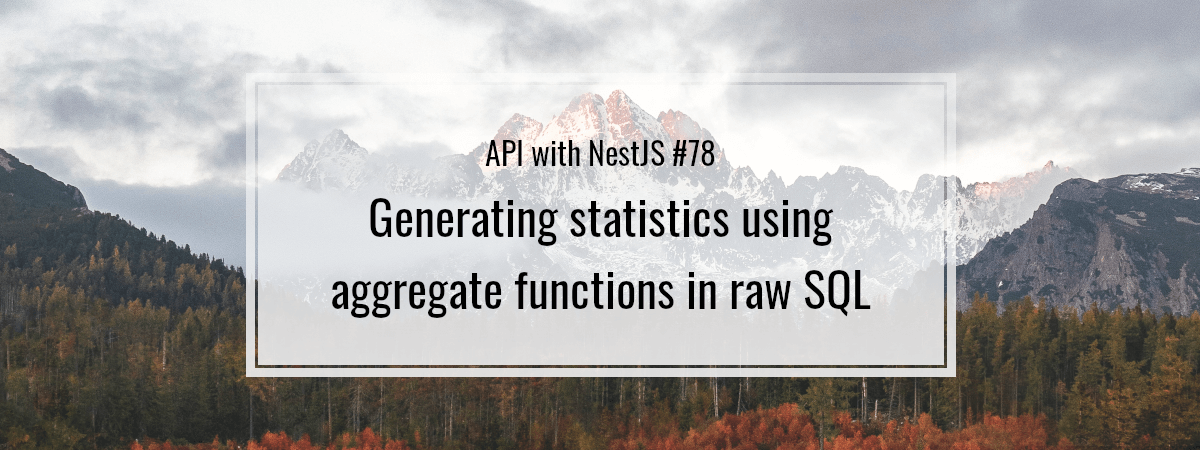This entry is part 78 of 125 in the API with NestJS

So far, we’ve been mostly writing SQL queries that either store or retrieve the data from the database. Besides that, we can rely on PostgreSQL to process the data and get the computed results. By doing that, we can learn more about the rows in our tables. In this article, we look into how we can use aggregate functions to generate statistics about our data.

## The purpose of aggregate functions

The job of an aggregate function is to compute a single result from multiple input rows. One of the most popular aggregate functions is . When used with an asterisk, it measures the total number of rows in the table.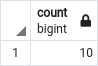When we provide the function with a column name, it counts the number of rows with a non-NULL value for that column.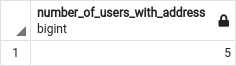The function was handy in the previous article when we implemented pagination.

## Grouping data in the table

Aggregate functions work great when we perform them on groups of data

When we do the above, PostgreSQL divides the data into groups and runs the aggregate function on each group individually.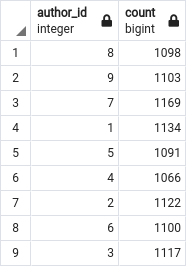We could make our query even more helpful and order our results. By doing that, we can ensure the authors with the highest number of posts are at the top of the list.

Let’s create a model that can hold the above data.

###### postAuthorStatistics.model.ts

Let’s create a separate statistics repository to prevent our class from getting too big.

###### postsStatistics.repository.ts

The function returns the value using the bigint data type. Because of that, we convert it to a regular integer. If you want to know more, check out the previous article.

We also need to point to our new repository in the class.

###### posts.service.ts

The last step is to use it in the controller.

###### posts.controller.ts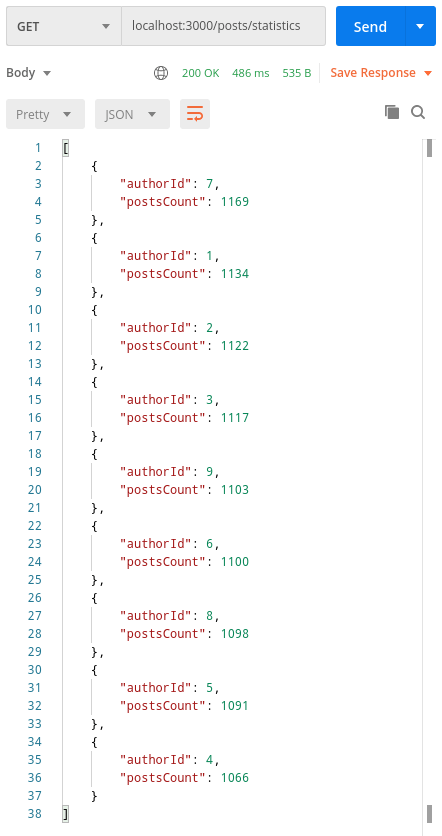## Other aggregate functions

There are more aggregate functions besides . Let’s go through them.

### max and min

Using the function, we can find the largest value of the selected column. Respectively, the function returns the smallest value of the column.

Since we don’t have any numerical columns in our , let’s pair the above functions with . This way, we can get the longest and shortest posts of a particular author.

The function returns the length of a string.

###### postsStatistics.repository.ts

Running or on a text column returns a string based on the alphabetical order.

### sum

With the function, we can return a total sum of a particular column. Since it only works with numerical values, we also need the  function.

Since the function also returns the value in the bigint format, we transform it to a regular integer. We can do it because we don’t expect values bigger than 2³¹⁻¹.

### avg

The function calculates the average of the values in a group. Let’s combine it with the function to calculate the average length of all posts of a particular author.

###### postsStatistics.repository.ts

A significant thing about the function is that it returns the data in the type. It can store many digits and is very useful when exactness is crucial. Parsing this data type to JSON converts it to a string by default. Since we don’t need many digits after the decimal, we convert it to the data type.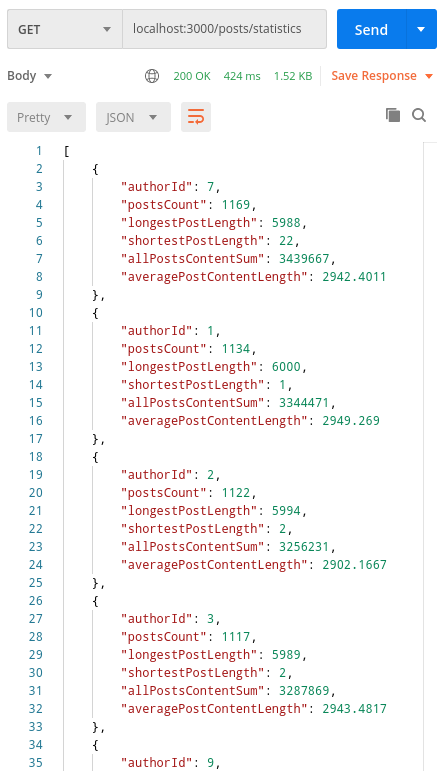## Aggregating data from more than one table

So far, we’ve been grouping and aggregating data in one table. However, a typical case might be when we want to aggregate data using more than one table. In this case, we need to use the keyword.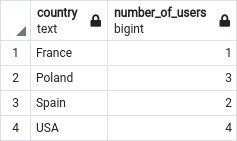Above, we perform an outer join using the keyword. If you want to know more, check out API with NestJS #73. One-to-one relationships with raw SQL queries

## Filtering using aggregate functions and grouping

So far, to filter the results from the database, we’ve been using the keyword.

When we want to filter using aggregate functions and grouping, we need to look at the execution order of SQL clauses.

Since PostgreSQL executes the clause before , we can’t use it with aggregate functions.

ERROR: aggregate functions are not allowed in WHERE
LINE 2: WHERE count(*) > 100

Instead, we need to use the keyword.

### Using aliases

An important caveat is that we can’t use column aliases with the keyword. So, for example, the following code wouldn’t work:

Instead, we need to use the function twice and rely on PostgreSQL to optimize it.

## Summary

In this article, we’ve gone through how to use aggregate functions together with grouping. When doing so, we’ve implemented an endpoint that returns statistics about a particular table. We also wrote an example that uses an aggregate function and grouping when joining two tables. Finally, we’ve also learned how to filter our data using aggregate functions and why we can’t do that with the keyword.

There is still more to cover when writing raw SQL with NestJS, so stay tuned!

Series Navigation<< API with NestJS #77. Offset and keyset pagination with raw SQL queriesAPI with NestJS #79. Implementing searching with pattern matching and raw SQL >>
Subscribe
Notify of